# Introduction

For the past month or so I’ve spent time digging into dynamic programming (hereby abbreviated to DP). There many great resources introducing the history and concepts of DP. However, what I wanted was the simplest example/demonstration of how DP works. Most examples were too convoluted for someone just learning it such as myself.

Luckily, while working through Advent of Code, I ran into a puzzle that led me down a rabbit hole to find the simplest example of DP. In this blog post I will introduce a problem, how we can brute force it, and then finally apply the DP algorithm to make it even better.

# The problem

“Given an array of numbers, find a contiguous subarray with the largest sum."

Let’s dive deeper into the problem. Suppose we are given an array of these numbers:

`[1, -2, 3, 5, -5]`

We want to find the largest sum of contiguous numbers. How do we approach this? Better yet, if I was to look at this problem and without attempting to use any code how would I find the answer? If we add all the numbers in the array, we would arrive at `2`, so any sub-sequence with a total sum larger than that could be the answer.

Let’s try again: we see that there is a `3` and a `5` equaling to `8`, in between 2 negative numbers. Upon a second scan, we realize that this is the answer:

The largest contiguous sequence is `[3, 5]` with a total sum of `8`.

##### A brute force solution

How would we go about solving this using code? Before anything else, let’s attempt to solve it using brute force. The idea here is that we would start with each number in the array and add the next number until we hit the end, and compare it to our max result. If we find that our current sum is greater than our max, we make our max the current sum. We do this continuously starting from the beginning to the end.

Let’s see what this looks like in action: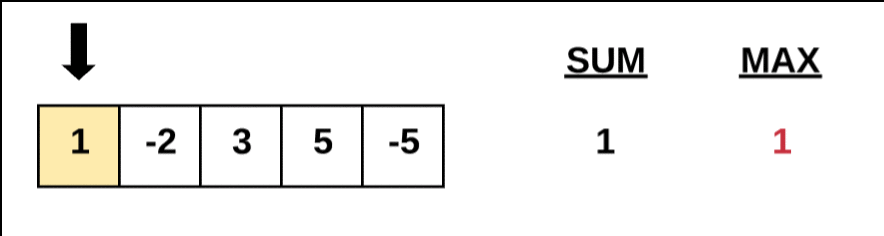And here’s what this looks like in code:

``````def max_sum_subarray(array):
max_sum = 0
array_len = len(array)

for i in range(array_len):
for j in range(i, array_len):
if i == j:
current_sum = array[i]
else:
current_sum = current_sum + array[j]

if current_sum > max_sum:
max_sum = current_sum

return max_sum
``````

As you can see, this is not very efficient. If we had a size `N` array, we are effectively looping through it `N` times. Our runtime is thus `O(N²)`, proportional to the size of our array. For large enough inputs, this will take a long time to complete.

Can we do better? Of course! (Or else this blog post wouldn’t exist.)

How do we go about making it better? If we look at our brute forced method, we see that we are, in fact, doing a lot of recalculations. What if we just save our previous best sum and compare them to our current sum?

This is in fact known as Kadane’s algorithm. What we are doing is still brute forcing, but saving the results of our previous calculations. In this way, we don’t have to iterate through the length of the array at every index. We only need to go through it once and comparing each sum to the previous one.

Our formula for deciding what sum to save is the max of our current item and our current item plus the previous sum:

`max(item, local_sum + item)`

Let’s walk through it: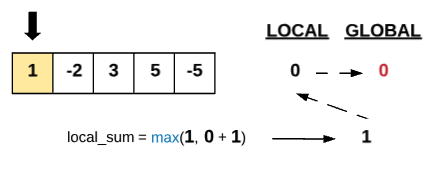We initialize two variables: a `global_sum` which will keep track of the maximum sum we’ve seen (akin to `max_sum` in the brute force) and a `local_sum` which keeps track of the last comparison.

We take the max of our current `item`, and our current `local_sum` plus our `item`. If the max happens to be just our item, we know our previous sum is not good enough to save so we start anew and save our current item as the best `local_sum`. Since our `local_sum` is now larger than our `global_sum`, we also save that.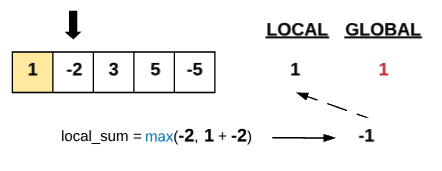Moving forward, we include the `-2` into our heuristic. We take the best of our current item (`-2`) and our our `local_sum` + current item. However, our local max sum is not as good as our global. Let’s keep going.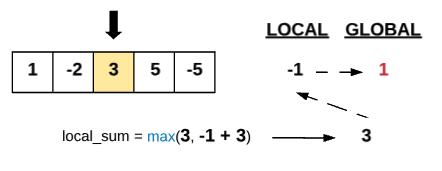Here things get a bit interesting. Our current item is actually larger than the `local_sum` + current item. This means that our previous continuous sum is not good enough so we start looking again with the `3` as our anchor. Since this new `local_sum` is also greater than our `global_sum`, we save it there as well.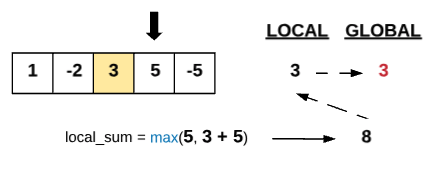Moving to our next item `5`, we notice that adding our current `local_sum` with our item gives us a larger sum than our previous global. This means we can add this to our running sum and also save this as our now largest `global_sum`.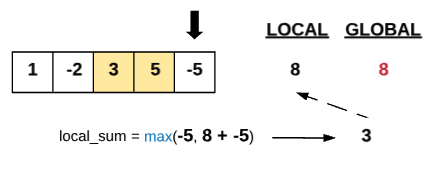Finally, we hit the last item in our array and it is somewhat underwhelming! The max `local_sum` we get here is `3` which is lower than our previous `local_sum` and our current `global_sum` so we can’t do anything with it. We then return our `global_sum` of `8`.

Let’s look at how this could be implemented:

``````def kadane(array):
global_sum = local_sum = 0

for item in array:
local_sum = max(item, local_sum + item)

if local_sum > global_sum:
global_sum = local_sum

return global_sum
``````

# Final thoughts

Our runtime is now linear in the number of items in the array. A great improvement. Of course, this is only the beginning. We can enhance this algorithm to include the start and ending indices of our maximum sum subarray by tracking when our `local_sum` is greater than our `global_sum`.

Throughout this walkthrough, I hope you noticed a certain pattern: at each step, we save the solution to our current subproblem, then come next round we check to see if what we previously calculated can be reused. And if so, we reuse it. If not, we scrap it and start fresh. This is the central theme to dynamic programming. This algorithm is surprisingly simple and elegant and demonstrates the power of saving previous computations.